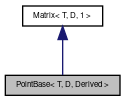MIRA
PointBase< T, D, Derived > Class Template Reference

The base template class of point, which covers the basic functionality of each point. More...

`#include <geometry/Point.h>`

Inheritance diagram for PointBase< T, D, Derived >:[legend]

## Public Types

enum  { Dim = D }

typedef T Type

typedef class Eigen::Map< Matrix, Aligned > AlignedMapType

typedef class Eigen::Map< Matrix, Unaligned > UnalignedMapType

## Public Member Functions

PointBase ()
Default constructor. More...

PointBase (const boost::geometry::model::point< T, D, boost::geometry::cs::cartesian > &other)
Create from boost geometry point. More...

template<typename DerivedMatrix >
PointBase (const Eigen::MatrixBase< DerivedMatrix > &other)
Create from Eigen matrix expression. More...

template<typename DerivedMatrix >
Derived & operator= (const Eigen::MatrixBase< DerivedMatrix > &other)
assignment of Eigen matrix expression More...

Derived & operator= (const boost::geometry::model::point< T, D, boost::geometry::cs::cartesian > &other)
converts from native boost::geometry::model::point More...

operator boost::geometry::model::point< T, D, boost::geometry::cs::cartesian > () const
converts to native boost::geometry::point More...

const Basebase () const

Basebase ()

const Scalar & coeff (int index) const

const Scalar & coeff (int row, int col) const

Scalar & coeffRef (int index)

Scalar & coeffRef (int row, int col)

int cols () const

Scalar * data ()

const Scalar * data () const

PacketScalar packet (int index) const

PacketScalar packet (int row, int col) const

void resize (int size)

void resize (int rows, int cols)

int rows () const

MatrixsetConstant (int rows, int cols, const Scalar &value)

MatrixsetConstant (int size, const Scalar &value)

MatrixsetIdentity (int rows, int cols)

MatrixsetOnes (int rows, int cols)

MatrixsetOnes (int size)

MatrixsetRandom (int rows, int cols)

MatrixsetRandom (int size)

MatrixsetZero (int rows, int cols)

MatrixsetZero (int size)

int stride (void) const

void swap (const MatrixBase< OtherDerived > &other)

void writePacket (int index, const PacketScalar &x)

void writePacket (int row, int col, const PacketScalar &x)

## Static Public Member Functions

static UnalignedMapType Map (Scalar *data, int rows, int cols)

static const UnalignedMapType Map (const Scalar *data, int rows, int cols)

static UnalignedMapType Map (Scalar *data, int size)

static const UnalignedMapType Map (const Scalar *data, int size)

static UnalignedMapType Map (Scalar *data)

static const UnalignedMapType Map (const Scalar *data)

static AlignedMapType MapAligned (Scalar *data, int rows, int cols)

static const AlignedMapType MapAligned (const Scalar *data, int rows, int cols)

static AlignedMapType MapAligned (Scalar *data, int size)

static const AlignedMapType MapAligned (const Scalar *data, int size)

static AlignedMapType MapAligned (Scalar *data)

static const AlignedMapType MapAligned (const Scalar *data)

## Protected Attributes

ei_matrix_storage< Scalar, MaxSizeAtCompileTime, RowsAtCompileTime, ColsAtCompileTime, Options > m_storage

## Detailed Description

### template<typename T, int D, typename Derived> class mira::PointBase< T, D, Derived >

The base template class of point, which covers the basic functionality of each point.

Basic functionality is type conversion, some constructors and operators. This class is a template which covers three parameters. The first parameter T gives the data type of the point, the second D gives the number of dimensions and the last parameter Derived represents the class which is derived from PointBase. Normally this class is for internal use only and the specialized point types should be sufficient. Anyway, it is not forbidden to use this template...

## ◆ Type

 typedef T Type

## ◆ anonymous enum

 anonymous enum
Enumerator
Dim

## ◆ PointBase() [1/3]

 PointBase ( )
inline

Default constructor.

## ◆ PointBase() [2/3]

 PointBase ( const boost::geometry::model::point< T, D, boost::geometry::cs::cartesian > & other )
inline

Create from boost geometry point.

## ◆ PointBase() [3/3]

 PointBase ( const Eigen::MatrixBase< DerivedMatrix > & other )
inline

Create from Eigen matrix expression.

## ◆ operator=() [1/2]

 Derived& operator= ( const Eigen::MatrixBase< DerivedMatrix > & other )
inline

assignment of Eigen matrix expression

## ◆ operator=() [2/2]

 Derived& operator= ( const boost::geometry::model::point< T, D, boost::geometry::cs::cartesian > & other )
inline

converts from native boost::geometry::model::point

## ◆ operator boost::geometry::model::point< T, D, boost::geometry::cs::cartesian >()

 operator boost::geometry::model::point< T, D, boost::geometry::cs::cartesian > ( ) const
inline

converts to native boost::geometry::point

The documentation for this class was generated from the following file: